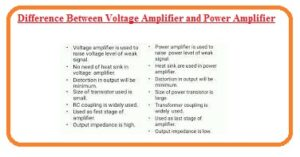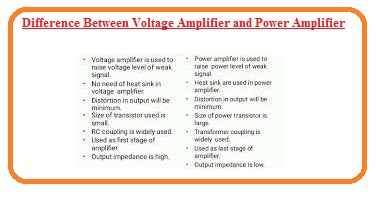Hello, friends I hope you all are doing great. In this post will have a detailed look at Difference Between Voltage Amplifier and Power Amplifier. The amplifier is circuitry that used to increase the signal level existing at the input and offers a high-value signal. The resulting signal called the applied signal and the process used called amplification.

There are 2 types of amplifiers first one is voltage and the second one power amplifier. The basic difference is that in voltage amplifier voltage level increases and in power amplifier power level of signal increases. so let get started with

## Difference Between Voltage Amplifier and Power Amplifier

What is Voltage Amplifier

• This type of circuit configuration do the voltage amplification and created in such configuration to increase the signal given at the input of circuitry.
• Its designing configuration relies at the maximum value of voltage gain.
• The gain is the ratio among the output to input and in the case of voltage amplifier, it is the ratio among the output voltage and input voltage.
• AV=Vo/p / Vip
• Let us assume that the voltage provided at input has one volt and output is twenty-five volts after the amplification process so its voltage gain will be twenty-five volts.
• Such amplifiers are such configured that very little quantity of power is given to the load.
• These appliers also called small-signal amplifiers.What is Power Amplifier

• The power amplifier is such circuitry configuration that created to increase the power level of the signal provided at the input of circuitry.
• To get a high value of power at the output there should be a high value of signal at the input.
• So there is the use of voltage amplifier at  input of the power amplifier.### Comparison between Voltage Amplifier and Power AmplifierVoltage Amplifier

• This amplifier used to increase the voltage level of the signal.
• It also called smaller signal amplifier configuration
• Its magnitude is less.
• The coupling circuit used for this circuit is RC.
• It used a smaller size base region.
• There is very less collector current for this circuitry.
• Its gain value is five to twenty.
• A small-size transistor is used for circuitry.
• Lad impudence value is four to ten-kilo ohm

Power amplifier

• This circuitry is used to increase the power level of the signal.
• Known as a large signal amplifier.
• Its magnitude is less.
• Tranfomerer could is used for this module.
• The base of the transistor has a larger area.
• Collector current is high almost one hundred ampers.
• The gain value is one hundred.
• Heat dissipation is large.
• Impedance is five to twenty ohms.

That is a detailed post about Difference Between Voltage amplifiers and Power amplifiers if you have any further query ask in comment. Thanks for reading.